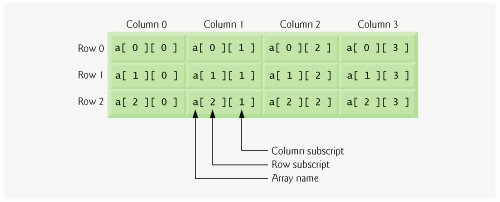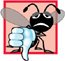# Multidimensional Arrays

Multidimensional arrays with two dimensions are often used to represent tables of values consisting of information arranged in rows and columns. To identify a particular table element, we must specify two subscripts. By convention, the first identifies the element's row and the second identifies the element's column. Arrays that require two subscripts to identify a particular element are called two-dimensional arrays or 2-D arrays. Note that multidimensional arrays can have more than two dimensions (i.e., subscripts). Figure 7.21 illustrates a two-dimensional array, a. The array contains three rows and four columns, so it is said to be a 3-by-4 array. In general, an array with m rows and n columns is called an m-by-n array.

Figure 7.21. Two-dimensional array with three rows and four columns.Every element in array a is identified in Fig. 7.21 by an element name of the form a[ i ][ j ], where a is the name of the array, and i and j are the subscripts that uniquely identify each element in a. Notice that the names of the elements in row 0 all have a first subscript of 0; the names of the elements in column 3 all have a second subscript of 3.

Common Programming Error 7.12Referencing a two-dimensional array element a[ x ][ y ] incorrectly as a[ x, y ] is an error. Actually, a[ x, y ] is treated as a[ y ], because C++ evaluates the expression x, y (containing a comma operator) simply as y (the last of the comma-separated expressions).

A multidimensional array can be initialized in its declaration much like a one-dimensional array. For example, a two-dimensional array b with values 1 and 2 in its row 0 elements and values 3 and 4 in its row 1 elements could be declared and initialized with

```int b[ 2 ][ 2 ] = { { 1, 2 }, { 3, 4 } };
```

The values are grouped by row in braces. So, 1 and 2 initialize b[ 0 ][ 0 ] and b[ 0 ][ 1 ], respectfully, and 3 and 4 initialize b[ 1 ][ 0 ] and b[ 1 ][ 1 ], respectfully. If there are not enough initializers for a given row, the remaining elements of that row are initialized to 0. Thus, the declaration

```int b[ 2 ][ 2 ] = { { 1 }, { 3, 4 } };
```

initializes b[ 0 ][ 0 ] to 1, b[ 0 ][ 1 ] to 0, b[ 1 ][ 0 ] to 3 and b[ 1 ][ 1 ] to 4.

Figure 7.22 demonstrates initializing two-dimensional arrays in declarations. Lines 1113 declare three arrays, each with two rows and three columns.

Figure 7.22. Initializing multidimensional arrays.

``` 1 // Fig. 7.22: fig07_22.cpp
2 // Initializing multidimensional arrays.
3 #include
4 using std::cout;
5 using std::endl;
6
7 void printArray( const int [][ 3 ] ); // prototype
8
9 int main()
10 {
11 int array1[ 2 ][ 3 ] = { { 1, 2, 3 }, { 4, 5, 6 } };
12 int array2[ 2 ][ 3 ] = { 1, 2, 3, 4, 5 };
13 int array3[ 2 ][ 3 ] = { { 1, 2 }, { 4 } };
14
15 cout << "Values in array1 by row are:" << endl;
16 printArray( array1 );
17
18 cout << "
Values in array2 by row are:" << endl;
19 printArray( array2 );
20
21 cout << "
Values in array3 by row are:" << endl;
22 printArray( array3 );
23 return 0; // indicates successful termination
24 } // end main
25
26 // output array with two rows and three columns
27 void printArray( const int a[][ 3 ] )
28 {
29  // loop through array's rows
30  for ( int i = 0; i < 2; i++ )
31  {
32  // loop through columns of current row
33  for ( int j = 0; j < 3; j++ )
34  cout << a[ i ][ j ] << ' ';
35
36  cout << endl; // start new line of output
37  } // end outer for
38 } // end function printArray
```

 ``` Values in array1 by row are: 1 2 3 4 5 6 Values in array2 by row are: 1 2 3 4 5 0 Values in array3 by row are: 1 2 0 4 0 0 ```

The declaration of array1 (line 11) provides six initializers in two sublists. The first sublist initializes row 0 of the array to the values 1, 2 and 3; and the second sublist initializes row 1 of the array to the values 4, 5 and 6. If the braces around each sublist are removed from the array1 initializer list, the compiler initializes the elements of row 0 followed by the elements of row 1, yielding the same result.

The declaration of array2 (line 12) provides only five initializers. The initializers are assigned to row 0, then row 1. Any elements that do not have an explicit initializer are initialized to zero, so array2[ 1 ][ 2 ] is initialized to zero.

The declaration of array3 (line 13) provides three initializers in two sublists. The sublist for row 0 explicitly initializes the first two elements of row 0 to 1 and 2; the third element is implicitly initialized to zero. The sublist for row 1 explicitly initializes the first element to 4 and implicitly initializes the last two elements to zero.

The program calls function printArray to output each array's elements. Notice that the function definition (lines 2738) specifies the parameter const int a[][ 3 ]. When a function receives a one-dimensional array as an argument, the array brackets are empty in the function's parameter list. The size of the first dimension (i.e., the number of rows) of a two-dimensional array is not required either, but all subsequent dimension sizes are required. The compiler uses these sizes to determine the locations in memory of elements in multidimensional arrays. All array elements are stored consecutively in memory, regardless of the number of dimensions. In a two-dimensional array, row 0 is stored in memory followed by row 1. In a two-dimensional array, each row is a one-dimensional array. To locate an element in a particular row, the function must know exactly how many elements are in each row so it can skip the proper number of memory locations when accessing the array. Thus, when accessing a[ 1 ][ 2 ], the function knows to skip row 0's three elements in memory to get to row 1. Then, the function accesses element 2 of that row.

Many common array manipulations use for repetition statements. For example, the following for statement sets all the elements in row 2 of array a in Fig. 7.21 to zero:

```for ( column = 0; column < 4; column++ )
a[ 2 ][ column ] = 0;
```

The for statement varies only the second subscript (i.e., the column subscript). The preceding for statement is equivalent to the following assignment statements:

```a[ 2 ][ 0 ] = 0;
a[ 2 ][ 1 ] = 0;
a[ 2 ][ 2 ] = 0;
a[ 2 ][ 3 ] = 0;
```

The following nested for statement determines the total of all the elements in array a:

```total = 0;

for ( row = 0; row < 3; row++ )

for ( column = 0; column < 4; column++ )
total += a[ row ][ column ];
```

The for statement totals the elements of the array one row at a time. The outer for statement begins by setting row (i.e., the row subscript) to 0, so the elements of row 0 may be totaled by the inner for statement. The outer for statement then increments row to 1, so the elements of row 1 can be totaled. Then, the outer for statement increments row to 2, so the elements of row 2 can be totaled. When the nested for statement terminates, total contains the sum of all the array elements.C++ How to Program (5th Edition)
ISBN: 0131857576
EAN: 2147483647
Year: 2004
Pages: 627